# Slope & Y-interceptPage 1

#### WATCH ALL SLIDES

Slide 1## Note

Note to browser’s

This slope lesson is interactive and I have students use graph paper as they follow along

This is formulated for the California High School Exit Exam math standards

Slide 2## Slope

Slide 3How do engineers build bridges?

Slide 4Slide 5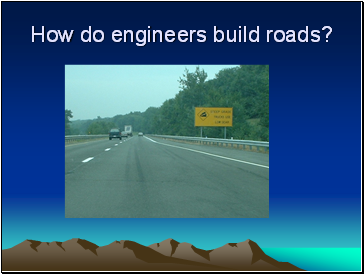Slide 6How can we write 7% as a fraction?

Slide 77% as a fraction??

Remember than any percent is a part of 100.

Slide 8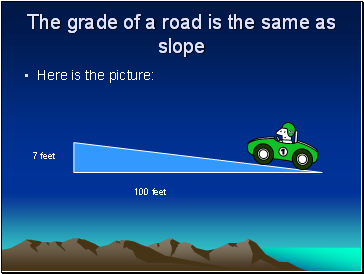Here is the picture:

7 feet

100 feet

Slide 9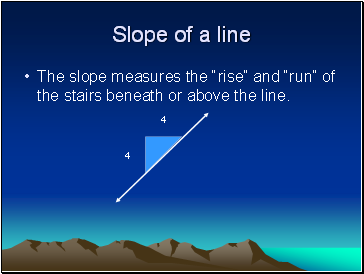## Slope of a line

The slope measures the “rise” and “run” of the stairs beneath or above the line.

4

4

Slide 10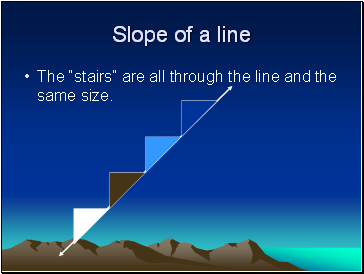Slope of a line

The “stairs” are all through the line and the same size.

Slide 11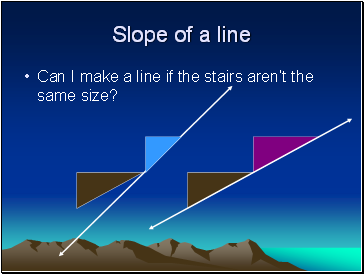Slope of a line

Can I make a line if the stairs aren’t the same size?

Slide 12Slope of a line (m)

To find the slope, use the formula

4

4

Slide 13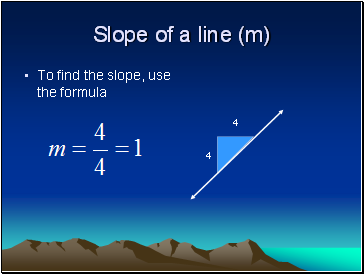Slope of a line (m)

To find the slope, use the formula

4

4

Slide 14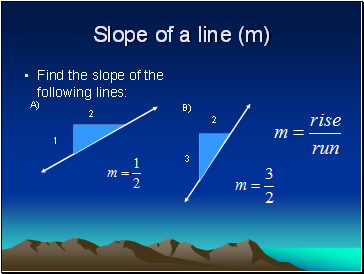Slope of a line (m)

Find the slope of the following lines:

1

2

A)

B)

2

3

Slide 15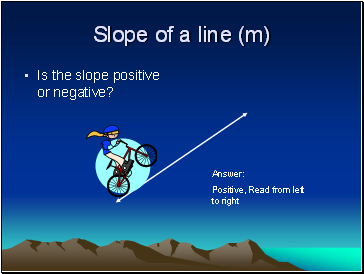Slope of a line (m)

Is the slope positive or negative?

Positive, Read from left to right

Slide 16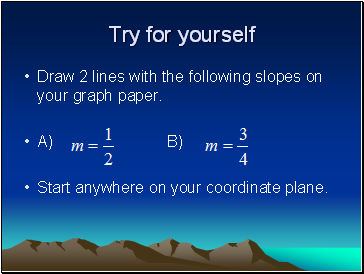Try for yourself

Draw 2 lines with the following slopes on your graph paper.

A) B)

Start anywhere on your coordinate plane.

Slide 17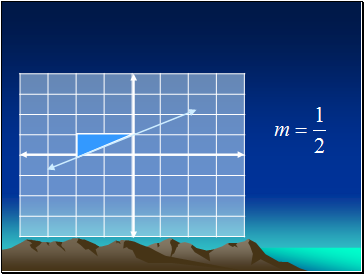Go to page:
1  2